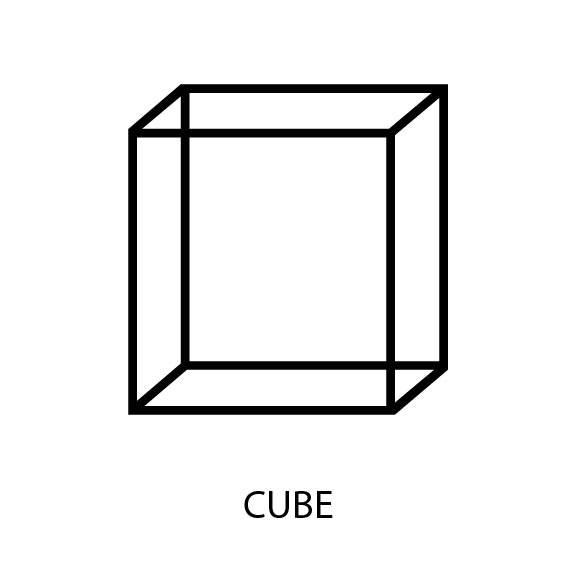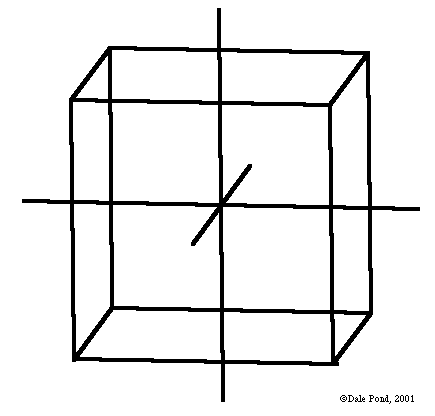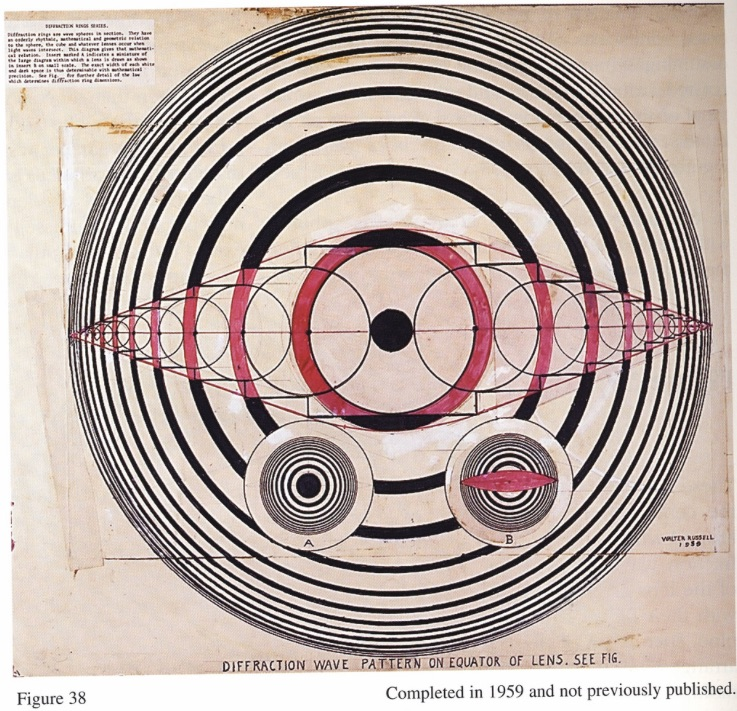# Cube

 CubeCube Sympsionics Symbol

noun: an object like a box with six square sides that are all the same size with all corners being square.
noun: the cube of a number is the larger number that is the result of multiplying the number by itself twice. For example, the cube of 2 is 2 x 2 x 2, which is 8.
Cubes have three planes, eight corners and volume.

Russell
"Step No. 5. One should now be able to fully comprehend that if one attempts to divide an unbalanced element like cobalt, by polarizing it, one will obtain an unbalanced result. Carbon, for example, is a balanced dual element. It is true cube and is white, while cobalt is distorted cube and is blue. The same experiment tried with carbon, or sodium-chloride, or potassium-bromide, would give balanced results. The ejections from both ends would be the same. If, on the contrary, sodium-iodide, or potassium-chloride is used the results would be as unbalanced as these latter pairs are unbalanced. Each of these pairs crystallize in distorted cubes while the evenly mated pairs crystallize in true cube, like carbon." [Atomic Suicide, page 276]Figure 6.3 - Cube with Orthogonal Vectors (click to enlarge)

Diffraction rings are wave spheres in sections. They have an orderly rhythmic, mathematical and geometric relation to the sphere, the cube and whatever lenses occur when light waves interact. This diagram gives that mathematical relation. Sphere marked A indicates a miniature of the large diagram within which a lens is drawn as shown in sphere B as small scale. The exact width of each white and dark space is thus determinable with mathematical precision.” [Dr. Walter Russell]

The formless zero universe is divided into four pairs of cubes and four pairs of spheres which are concentrically placed within each other. These four pairs of cube-spheres constitute one tonal octave of the universal harp. These tonal octaves are eight in number by appear to be nine for the first half of the ninth octave is the last half of the first. [Geometry and Mathematics of Octave Waves]

"The formless zero universe is divided into four pairs of cubes and four pairs of spheres which are concentrically placed within each other. These four pairs of cube-spheres constitute one tonal octave of the universal harp. These tonal octaves are eight in number but appear to be nine for the first half of the ninth octave is the last of the first." [zero universe](courtesy University of Science and Philosophy) (click to enlarge)

Ramsay
"The ratio of 1:2 is essentially simple in its character, and any power of the prime 2 always produces a note like itself. It is a law in musical science that doubling or halving a number never changes its character. Whatever ratios and notes are produced from the first power, the square, and the cube of any number, the same kind of ratios and notes will be produced, in the genesis of octaves, by the doubles or halves of that number. On this account the prime 2 has unlimited powers in producing notes, and is used in the first place in getting a series of octaves from 1 as unity;" [Scientific Basis and Build of Music, page 26]

"While the ratio of 1:2 corresponds to rest, and to the force of gravity, the ratio of 2:3 corresponds to motion, and to the centrifugal force. The prime 2, by any of its powers, never produces a new note. The prime 3 always produces a new note, and on this account its powers are limited to the first power, the square, and the cube, and each of these powers of 3 produces one new note." [Scientific Basis and Build of Music, page 27]

When we have got F1, and from it C3 and A5 by the primes of 3 and 5 multiplying 1, then all the octaves of the these three notes will be found by the prime 2, multiplying by it for the higher, and dividing by it for the lower octaves. When from C3 we have got G9 and E15, multiplying by the primes 3 and 5, then the octaves of these are also found by the prime 2, used again in the same way. And when from G9 we have got D27 and B45 by the primes 3 and 5, the octaves of these are also found by the prime 2. The prime 2 has an unlimited use; the prime 3 is used in the first power, the square, and the cube; the prime 5 in the first power only. Thus is evolved the true major scale, and no need for a B♭ or any other tinkering. [Scientific Basis and Build of Music, page 31]